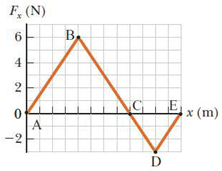# Problem: The force acting on a particle varies as in the figure below. (The x axis is marked in increments of 1.50 m.)Find the work done by the force as the particle moves from x = 12.0 m to x = 18.0 m

###### FREE Expert Solution

The work done is equal to the area under the curve.

x = 12.0 m to x = 18.0 m refers to triangle CDE.

85% (88 ratings)###### Problem Details

The force acting on a particle varies as in the figure below. (The x axis is marked in increments of 1.50 m.)

Find the work done by the force as the particle moves from x = 12.0 m to x = 18.0 m